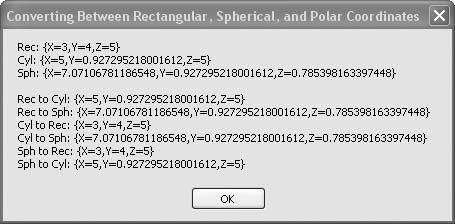# Recipe 6.15. Converting Between Rectangular, Spherical, and Cylindrical Coordinates

#### Problem

You need to convert three-dimensional coordinates between rectangular, spherical, and cylindrical notation.

#### Solution

Sample code folder: Chapter 06\Convert3D

Create a set of six functions to convert Point3D variables to and from each coordinate notation.

#### Discussion

The following six functions convert from any one of the three types of three-dimensional coordinates to any of the others. All these functions accept a Point3D argument and return a Point3D value. It is up to you to keep track of the current type of coordinate notation in each Point3D variable. Note that in all cases the Point3D value passed in to any of these functions is not altered; a new Point3D instance is returned instead. Here are the six functions:

` Public Function RectToCylinder(ByVal pointA As Point3D) _       As Point3D    ' ----- Convert   rectangular 3D coordinates to    '       cylindrical coordinates.    Dim rho As Double    Dim theta As Double    rho = Math.Sqrt(pointA.X ^ 2 + pointA.Y ^ 2)    theta = Math.Atan2(pointA.Y, pointA.X)    Return New Point3D(rho, theta, pointA.Z) End Function Public Function CylinderToRect(ByVal pointA As Point3D) _       As Point3D    ' ----- Convert cylindrical coordinates to    '         rectangular 3D coordinates.    Dim x As Double    Dim y As Double    x = pointA.X * Math.Cos(pointA.Y)    y = pointA.X * Math.Sin(pointA.Y)    Return New Point3D(x, y, pointA.Z) End Function Public Function RectToSphere(ByVal pointA As Point3D) _       As Point3D    ' ----- Convert rectangular 3D coordinates to    '         spherical coordinates.    Dim rho As Double    Dim theta As Double    Dim phi As Double    rho = Math.Sqrt(pointA.X ^ 2 + pointA.Y ^ 2 + _       pointA.Z ^ 2)    theta = Math.Atan2(pointA.Y, pointA.X)    phi = Math.Acos(pointA.Z / Math.Sqrt( _       pointA.X ^ 2 + pointA.Y ^ 2 + pointA.Z ^ 2))    Return New Point3D(rho, theta, phi) End Function Public Function SphereToRect(ByVal pointA As Point3D) _       As Point3D    ' ----- Convert spherical coordinates to    '       rectangular 3D coordinates.    Dim x As Double    Dim y As Double    Dim z As Double    x = pointA.X * Math.Cos(pointA.Y) * Math.Sin(pointA.Z)    y = pointA.X * Math.Sin(pointA.Y) * Math.Sin(pointA.Z)    z = pointA.X * Math.Cos(pointA.Z)    Return New Point3D(x, y, z) End Function Public Function CylinderToSphere(ByVal pointA As Point3D) _       As Point3D    ' ----- Convert cylindrical   coordinates to    '         spherical coordinates.    Dim rho As Double    Dim theta As Double    Dim phi As Double    rho = Math.Sqrt(pointA.X ^ 2 + pointA.Z ^ 2)    theta = pointA.Y    phi = Math.Acos(pointA.Z / _       Math.Sqrt(pointA.X ^ 2 + pointA.Z ^ 2))    Return New Point3D(rho, theta, phi) End Function Public Function SphereToCylinder(ByVal pointA As Point3D) _       As Point3D    ' ----- Convert spherical coordinates to    '       cylindrical coordinates.    Dim rho As Double    Dim theta As Double    Dim z As Double    rho = pointA.X * Math.Sin(pointA.Z)    theta = pointA.Y    z = pointA.X * Math.Cos(pointA.Z)    Return New Point3D(rho, theta, z) End Function `

The following code creates several Point3D variables using names that indicate the types of coordinates they contain. For example, pointCyl is a Point3D variable containing three-dimensional cylindrical coordinates. The various conversion functions are used to populate the variables, and the results are shown in Figure 6-15:

` Dim result As New System.Text.StringBuilder Dim pointRec As New Point3D(3, 4, 5) Dim pointCyl As Point3D = RectToCylinder(pointRec) Dim pointSph As Point3D = RectToSphere(pointRec) Dim pointRecToCyl As Point3D = RectToCylinder(pointRec) Dim pointRecToSph As Point3D = RectToSphere(pointRec) Dim pointCylToRec As Point3D = CylinderToRect(pointCyl) Dim pointCylToSph As Point3D = CylinderToSphere(pointCyl) Dim pointSphToRec As Point3D = SphereToRect(pointSph) Dim pointSphToCyl As Point3D = SphereToCylinder(pointSph) result.AppendLine("Rec: " & pointRec.ToString()) result.AppendLine("Cyl: " & pointCyl.ToString()) result.AppendLine("Sph: " & pointSph.ToString()) result.AppendLine() result.AppendLine("Rec to Cyl: " & pointRecToCyl.ToString()) result.AppendLine("Rec to Sph: " & pointRecToSph.ToString()) result.AppendLine("Cyl to Rec: " & pointCylToRec.ToString()) result.AppendLine("Cyl to Sph: " & pointCylToSph.ToString()) result.AppendLine("Sph to Rec: " & pointSphToRec.ToString()) result.AppendLine("Sph to Cyl: " & pointSphToCyl.ToString()) MsgBox(result.ToString()) `

##### Figure 6-15. Converting Point3D variables between three different types of spatial coordinatesSearch for " rectangular cylindrical spherical" on the Web for a variety of explanations and further information about this subject.Visual Basic 2005 Cookbook: Solutions for VB 2005 Programmers (Cookbooks (OReilly))
ISBN: 0596101775
EAN: 2147483647
Year: 2006
Pages: 400

Similar book on Amazon